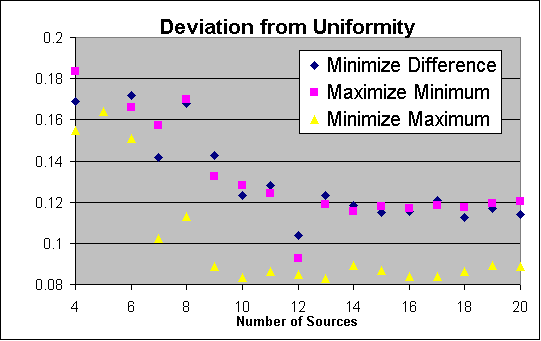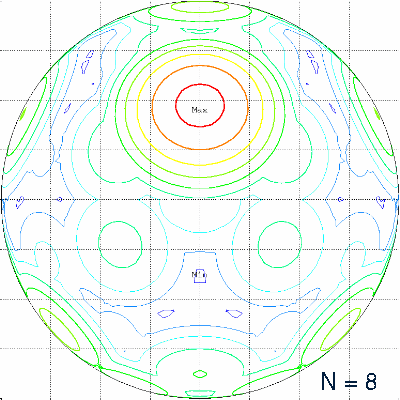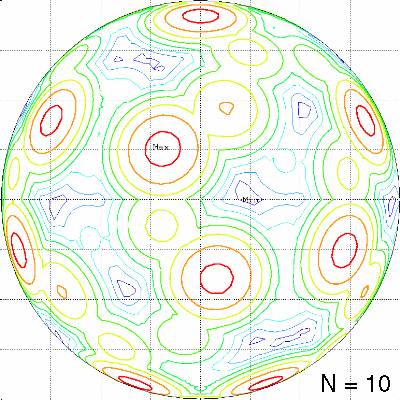## Deviation from uniform illumination as a function of N

An ideal solution would have a constant luminance of N/4 over the whole surface of the target sphere. The following figure shows a comparison of the best solutions achieved so far for the three different criteria.
Criterion
Value
Minimize difference:
(Imax - Imin) / 2
Maximize minimum:
N/4 - Imin
Minimize maximum:
Imax - N/4For N=5 only the "minimize maximum" results are shown, because the other two results would require an extension of the scale (0.51787/2=0.25893 for "minimize difference" and 1.25-0.93969=0.31031 for "maximize minimum").

### Character of the optimal solutions

The character of the optimal solutions is different, dependent on the applied criterion. A typical property of the "minimize maximum" configurations is the occurrence of at least 2N luminance maxima with a smooth behavior in the neighbourhood of the maxima. As the value of the minimum luminance has no influence on the objective function, there are often a few "dark holes" in the optimal luminance pattern, as can be seen in the lower half of the following picture:The optimal "maximize minimum" configurations have large regions of low luminance with minima at locations of discontinuity in the local luminance gradient. There are a few (often 2) bright spots, again caused by their missing influence on the chosen objective function. See e.g. the following picture:The solutions for the criterion "minimize difference" avoid the preference for either minima or maxima. The corresponding optimal configurations produce luminance distributions with comparable numbers of mimima and maxima, as can be seen in the following example: